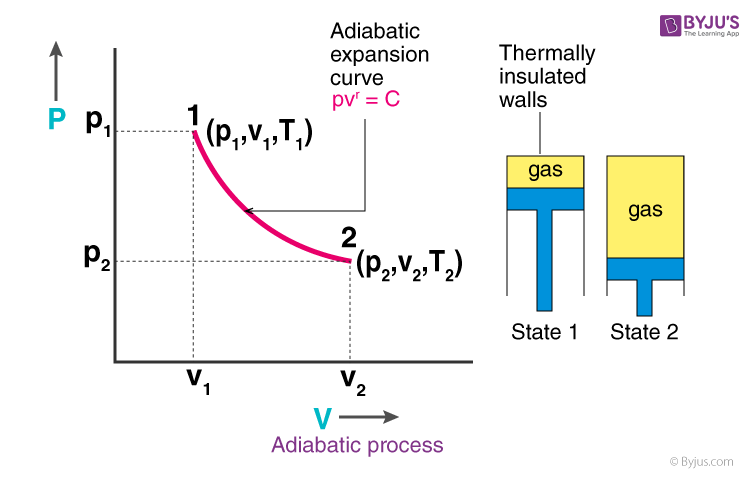As we know, heat, temperature, and the external environment are factors responsible for the flow of heat from one system to another. Changes do take place when heat is transferred. A process in which no heat transfer takes place is known as an adiabatic process. In this article, let us understand in detail the adiabatic process with examples.

An adiabatic process is defined as

The thermodynamic process in which there is no exchange of heat from the system to its surrounding neither during expansion nor during compression.

The adiabatic process can be either reversible or irreversible. Following are the essential conditions for the adiabatic process to take place:

• The system must be perfectly insulated from the surrounding.
• The process must be carried out quickly so that there is a sufficient amount of time for heat transfer to take place.For instance, the gas compression within an engine cylinder is expected to happen so fast that on the compression process timescale, a minimum amount of the energy of the system could be produced and sent out in the form of heat.

Despite the cylinders being not insulated and having a conductive nature, the process is deemed to be adiabatic. The same could be considered to be true for the enlargement process of such a system.

### What is Adiabatic Process Equation?

Following is the adiabatic process equation:

 PVγ = constant

Where,

• P is the pressure of the system
• V is the volume of the system
• γ is the adiabatic index and is defined as the ratio of heat capacity at constant pressure Cp to heat capacity at constant volume Cv

The reversible adiabatic process is also called an  Isentropic Process. It is an idealized thermodynamic process that is adiabatic and in which the work transfers of the system are frictionless; there is no transfer of heat or of matter, and the process is reversible. Such an idealized process is useful in engineering as a model and basis of comparison for real processes.

Adiabatic expansion is defined as the expansion in which there is no heat interaction of the system with the surroundings and work is done by the system at the expense of its internal energy.

Adiabatic compression of the air is defined as the compression in which no heat is added or subtracted from the air, and the internal energy of the air is increased, which is equal to the external work done on the air. The pressure of the air is more than the volume as the temperature increases during compression.

There are several instances, some are stated below:

• It is a process where there is a gas compression and heat is generated. One of the simplest examples would be the release of air from a pneumatic tire.
• Adiabatic Efficiency is applied to devices such as nozzles, compressors, and turbines. One of the good applications of the adiabatic process.
• The pendulum oscillating in a vertical plane is an example of it.
• A quantum harmonic oscillator is also an example of an adiabatic system.
• When we put the ice into the icebox, no heat goes out and no heat comes in.

## Difference Between Isothermal and Adiabatic Process

Following is the table explaining the isothermal V/s adiabatic process:

 Isothermal process Adiabatic process An isothermal process is defined as one of the thermodynamic processes which occur at a constant temperature An adiabatic process is defined as one of the thermodynamic processes which occur without any heat transfer between the system and the surrounding Work done is due to the change in the net heat content in the system Work done is due to the change in its internal energy The temperature cannot be varied The temperature can be varied There is a transfer of heat There is no transfer of heat

In the video, the expression for the adiabatic process is derived and explained.## Frequently Asked Questions – FAQs

The adiabatic process is a thermodynamic process in which there is no heat transfer from in or out of the system. An adiabatic process is a reversible process with constant entropy for an ideal gas. The mathematical representation of the adiabatic process is ΔQ=0.

Adiabatic expansion is defined as the expansion in which there is no heat interaction of the system with the surroundings and work is done by the system at the expense of its internal energy.